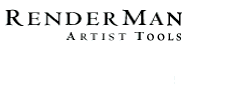PhotoRealistic RenderMan
Application Note #19

March, 1997

Introduction

Beginning with PhotoRealistic RenderMan 3.7, a new RiCurves primitive has been added which provides a mechanism for specifying 3-D curves. One may think of this primitive as a ribbon or a string of spaghetti. It is efficient to render large numbers of these primitives, so it is ideally suited to modeling such things as hair and grass.

Syntax

RiCurves( RtToken type, RtInt ncurves, RtInt nvertices[], RtToken wrap, parameterlist );

RiCurvesV( RtToken type, RtInt ncurves, RtInt nvertices[], RtToken wrap,
RtInt n, RtToken tokens[], RtPointer parms[] );

Draws one or more 3-D ribbons of specified width through a set of control vertices. Multiple disconnected individual curves may be specified using one call to RiCurves. The parameter ncurves is the number of individual curves specified by this command, and nvertices is an array of length ncurves integers specifying the number of vertices in each of the respective curves.

The interpolation method given by type can be either "linear" or "cubic" (note: not "bilinear" or "bicubic"). Cubic curves interpolate using the v basis matrix and step size set by RiBasis. The u parameter changes across the width of the curve, while the v parameter changes across the length of the curve (i.e. the direction specified by the control vertices).

Curves may wrap around in the v direction, depending on whether wrap is "periodic" or "nonperiodic". Curves which wrap close upon themselves at the ends and the first control points will be automatically repeated. As many as three control points may be repeated, depending on the basis matrix of the curve.

parameterlist is a list of token-value pairs where each token is one of the geometric primitive variables or a variable that has been defined with RiDeclare. The parameter list must include at least position ("P" or "Pw") information. The width along the curve may be specified with either a special "width" parameter which is a varying float argument, or a "constantwidth" parameter which is a constant float (one value for the entire RiCurves). Widths are specified in object space units of the curve. If no "width" vector or "constantwidth" value is given, the default width is 1.0 units in object space.

Since an RiCurve generates a flat ribbon, but the control vertices only specify the direction of the "spine", the rotation of the flat ribbon about the spine is ambiguous. If no normals ("varying normal N") are specified in the parameter list, the ribbon will rotate so that it is as perpendicular to the view plane as possible. This is a good way to simulate a thin tube, since the silhouette of the ribbon will match that of the tube. However, if "N" values are supplied, the normals will be used to guide the ribbon so that it stays perpenticular to the supplied normals, thus allowing user-controlled rotation of the ribbon. To summarize, if you supply "N" values, you will get something like grass, and if you do not supply "N" values, you will get something like hair.

The number of data items required for constant, uniform, varying, or vertex parameters for curves is as follows:

constant:

one single value for the entire RiCurves primitive.
uniform:
ncurves values
varying:
sum (nsegsi+1) values for nonperiodic curves,
sum (nsegsi) values for periodic curves.
vertex:
sum(nvertices[i])

where sum() indicates summing over all the individual curves specified by the RiCurves statement, and:

nsegsi is the number of segments in curve #i
= nvertices[i]-1 for linear, nonperiodic curves
= nvertices[i] for linear, periodic curves
= (nvertices[i]-4)/vstep+1 for cubic, nonperiodic curves
= nvertices[i]/vstep for cubic, periodic curves

RIB BINDING

Curves type [nvertices] wrap parameterlist

EXAMPLE

Curves "cubic"  "nonperiodic" "P" [0 0 0 -1 -.5 1 2 .5 1 1 0 -1 ] "width" [.1 .04]

Curves "linear"  "nonperiodic" "P" [0 0 0 3 4 5 -1 -.5 1 2 .5 1 1 0 -1 ] "constantwidth" [0.075]

Using RiCurves for Hair

PhotoRealistic RenderMan 3.7 is able to render large numbers (hundreds of thousands or more) of curves very efficiently, making this primitive particularly well suited for modeling hair. Since the default behavior (when normals are not supplied) is to generate ribbons that keep their flat side facing the camera, we are creating geometry which has the same silhouette as a hair, but is perhaps hundreds of times more effiencient to render (in both time and memory) than an equivalent surface of circular cross section.

But the hairs are just ribbons, not really round tubes. If we light them using a standard illumination model (like plastic.sl), they will not look like hair. Flat ribbons don't reflect light the same way that a tube does. But we can make the illusion complete by writing a shader for use with the curves which reacts to light as if it was a thin cylinder.

surface hair (float Ka = 1, Kd = .6, Ks = .35, roughness = .15;
color rootcolor = color (.109, .037, .007);
color tipcolor = color (.519, .325, .125);
color specularcolor = (color(1) + tipcolor) / 2;
)
{
vector T = normalize (dPdv); /* tangent along length of hair */
vector V = -normalize(I);    /* V is the view vector */
color Cspec = 0, Cdiff = 0;  /* collect specular & diffuse light */
float cosang;

/* Loop over lights, catch highlights as if this was a thin cylinder */
illuminance (P) {
cosang = cos (abs (acos (T.normalize(L)) - acos (-T.V)));
Cspec += Cl * v * pow (cosang, 1/roughness);
Cdiff += Cl * v;
/* We multipled by v to make it darker at the roots.  This
* assumes v=0 at the root, v=1 at the tip.
*/
}

Oi = Os;
Ci = Oi * (mix(rootcolor, tipcolor, v) * (Ka*ambient() + Kd*Cdiff)
+ (Ks * Cspec * specularcolor));
}

Below is an example showing what RiCurve primitives look like, in combination with the shader above: